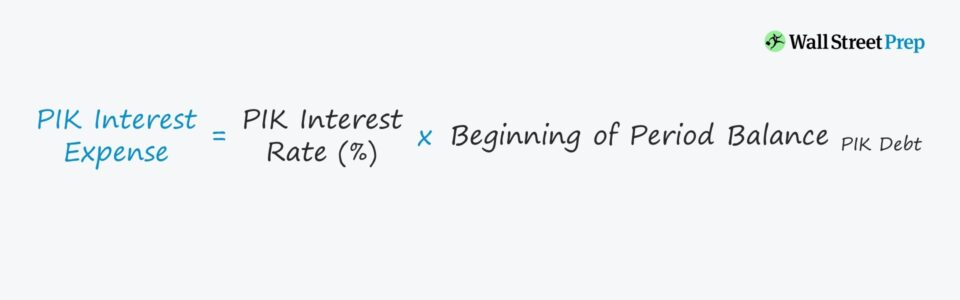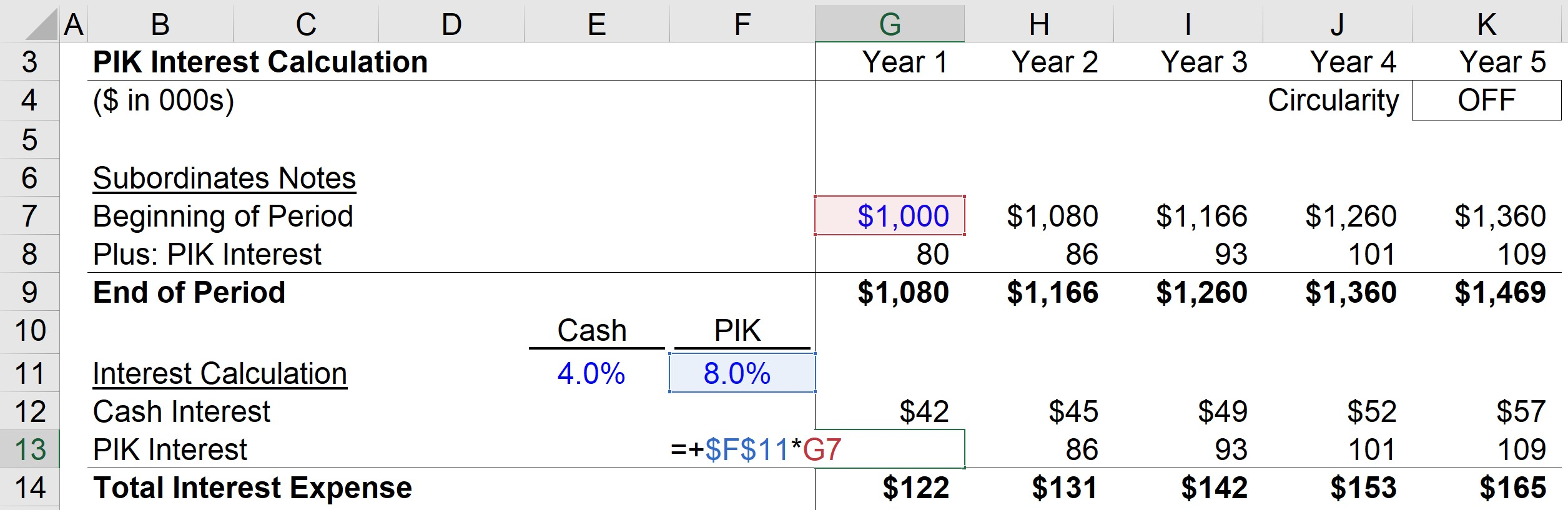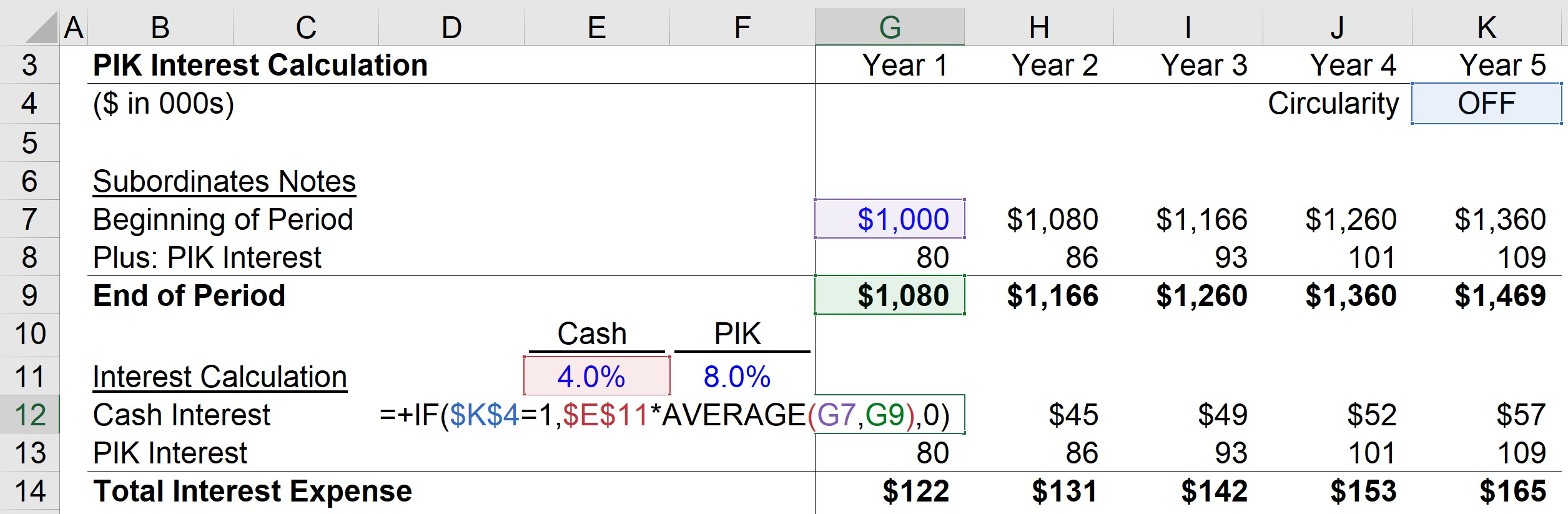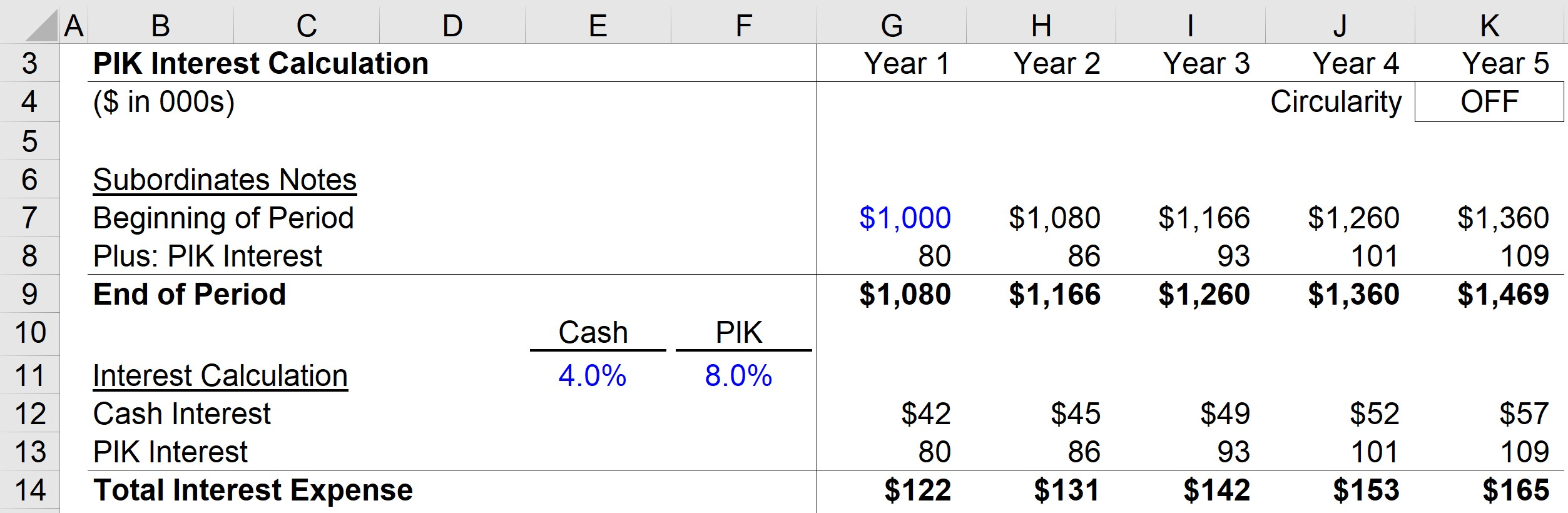Welcome to Wall Street Prep! Use code at checkout for 15% off.# PIK Interest

Guide to Understanding PIK Interest## How to Calculate PIK Interest?

PIK interest stands for “Paid-in-Kind” and is defined as the amount of interest expense charged by a lender which accrues towards the ending debt balance (principal).

Opting for PIK helps the borrower conserve cash since the interest payments are pushed back to a later date. Or in the case of preferred equity, the payout of cash dividends could be deferred for a set, agreed-upon duration.

The downside to the accrued interest, however, is that the total debt principal increases each year until maturity. In effect, this increases the interest expense due to growth in the principal amount.

With each passing period, the amount of accrued interest due can accumulate quickly due to the effects of compounding interest, which can significantly heighten default risk.

## PIK Accrual: Compounding Interest Expense

PIK interest benefits the borrower by providing the optionality to push back cash interest payments on debt.

In turn, lenders are compensated by the accrual of the periodic interest expense towards the ending balance (i.e. higher principal) until maturity.

The PIK rate also typically accrues at a rate higher than the cash interest rate in lieu of immediate cash compensation.

Each year after the issuance of a PIK security, the interest expense owed is impacted by the following factors:

1. Initial Principal Amount
2. “Rolled-Up” Interest

Certain debt instruments may come with a partial PIK component. For example, a loan with a 10.0% interest rate and 50.0% PIK component means that half of the interest is going to be paid using cash, while the remaining half is accrued.

## PIK Interest Formula

To calculate the paid-in-kind interest, the formula consists of the PIK rate being multiplied by the beginning balance of the applicable debt security or preferred equity.

PIK Interest = PIK Interest Rate (%) x Beginning of Period Balance of PIK Debt

Note that if there are mandatory repayments (i.e. principal amortization) associated with the debt, the formula must account for the repaid debt.

This would reduce the interest expense due and the end-of-period debt balance.

Whether the interest expense is paid in cash or PIK, the debt principal and the accrued interest payments must be paid by maturity at the end of the borrowing term, per the lending agreement.

## How to Model the PIK Toggle?

Often, debt comes arranged with a fixed PIK schedule outlined in the lending agreement.

But another form of PIK interest is referred to as a PIK toggle, which is an agreement between the issuer and borrower that provides the borrower with the option to defer an interest payment if needed.

Based on the liquidity needs of the borrower (i.e. cash on hand) or other conditional provisions, this feature lets a borrower reduce its cash outflows.

If a PIK toggle is in place, the decision on whether interest expense is paid-in-cash or PIK becomes more of a discretionary decision made on the specific circumstances regarding the credit health of the borrower.

PIK interest can be especially attractive to borrowers that are looking to avoid having to incur interest payments to conserve cash (i.e., leveraged buyouts).

In addition, companies that have found themselves in poor financial conditions and in need of debt restructuring can seek to renegotiate debt terms to include the option for PIK.

## Is PIK Interest Tax Deductible?

To confirm your understanding of the PIK interest concept, review the following accounting question.

If a company has incurred \$10 in PIK interest, how are the three financial statements impacted?

• I/S: On the income statement, interest expense will increase by \$10, which causes net income to decrease by \$7 given a 30% tax rate assumption.
• CFS: On the cash flow statement, net income will be down by \$7, but the \$10 non-cash PIK interest is added back. The ending cash balance will reflect an increase of \$3.
• B/S: On the assets side of the balance sheet, cash will have increased by \$3. Then on the liabilities & equity side, the debt balance should have increased by \$10 since the PIK accrues to the debt’s ending balance, and net income is going to be down by \$7. Putting them together, both the assets and liabilities & equity side is up by \$3 (and the balance sheet remains in balance).

## How to Adjust Coverage Ratio for PIK Interest?

Note that lending agreements occasionally include interest expense to be paid in the form of “paid-in-kind” interest (or PIK interest), rather than cash interest.

In such cases, an interest expense coverage ratios can be adjusted to exclude the effects of any PIK interest.

In effect, only the cash portion of interest expense should be included in the calculation, because PIK is not an actual outflow of cash.

All else being equal, PIK interest increases interest coverage ratios since they are not counted as part of the “interest” line, but note that interest is still accruing to the debt principal and is due at maturity.

## PIK Interest Calculator

We’ll now move to a modeling exercise, which you can access by filling out the form below.Submitting...

## 1. Subordinated Notes Assumptions

Suppose we’re tasked with forecasting the interest expense of a hypothetical company that has borrowed subordinated notes with the PIK optionality.

The debt assumptions we’ll use for this modeling exercise are listed below.

• Subordinates Notes, Beginning Balance (Year 1) = \$1m
• PIK Interest Rate = 8.0%
• Cash Interest Rate = 4.0%

Rather than a straight 12.0% cash interest rate, 4.0% will be paid-in-cash with 8.0% charged in the form of PIK – meaning that throughout the borrowing period, the 8.0% PIK interest accrues towards the beginning balance.

## 2. PIK Interest Calculation Example

In Year 1, the beginning balance of \$1m is multiplied by the 8.0% PIK rate to calculate the interest expense, which comes out to be \$80k.

Therefore, we can see how the \$80k of interest accrued towards the principal for the ending balance calculation for Year 1 for a total of \$1.08m.Here, we can see the direct impact that accrued interest (and the increased balance) has on the amount of interest due each period; or put differently, the compounding effects of PIK interest.

For comparison, we’ll calculate the portion of interest expense paid in cash by multiplying the interest rate (4.0%) by the average subordinated notes balance.

Interest Expense = Interest Rate x Average (Beginning, Ending Debt Balance)

And since using the average balance in the interest expense formula introduces a circularity into our model, we’ll add a circuit breaker.

• OFF: If the circularity cell (\$K\$4) is set to 1, the circuit breaker is turned off
• ON: Or if a zero is entered into the cell, the circuit breaker is switched on, and the output will be zero (i.e. cutting off the circularity-inducing calculation)

For instance, the Year 1 cash interest expense is equal to the 4.0% cash interest rate multiplied by the average of the beginning and ending Year 1 sub-notes balance (\$1m and \$1.08m). This comes out to \$42k for the cash interest payment in Year 1.If the cash interest component did not exist and the form of interest was instead PIK, no cash interest would be paid throughout the term of the loan.

## 3. Accrued Interest Analysis and Ending Debt Principal Calculation

Once the debt matures, the borrower must repay the original debt principal and all the accrued interest.

But in our simplified example, the subordinated notes balance at the end of each period is equal to the sum of the PIK beginning balance and PIK interest accrued.

So in closing, the principal of the subordinated notes has reached approximately \$1.47m by the end of Year 5 from the initial balance of \$1m at the beginning of Year 1.Step-by-Step Online Course

### Everything You Need To Master Financial Modeling

Enroll in The Premium Package: Learn Financial Statement Modeling, DCF, M&A, LBO and Comps. The same training program used at top investment banks.Inline FeedbacksLearn Financial Modeling Online

Everything you need to master financial and valuation modeling: 3-Statement Modeling, DCF, Comps, M&A and LBO.

X

The Wall Street Prep Quicklesson Series

7 Free Financial Modeling Lessons

Get instant access to video lessons taught by experienced investment bankers. Learn financial statement modeling, DCF, M&A, LBO, Comps and Excel shortcuts.